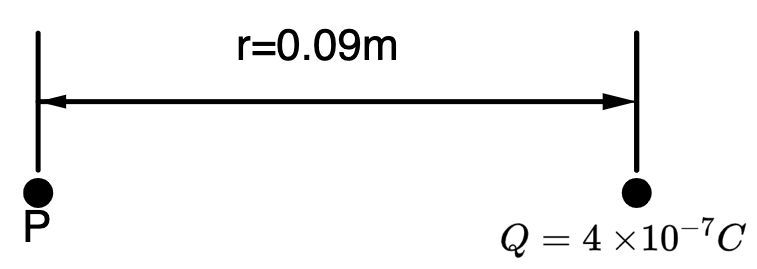Home/Class 12/Physics/

## QuestionPhysicsClass 12

Calculate the potential at a point  $$P$$  due to a charge of  $$4\times 10^{-7}C$$  located  $$9\text{ cm }$$ away.

$$4\times {10}^{4}\ V$$
4.64.6## Solution

Given data,
Charge,$$Q=4\times 10^{-7}C$$
Distance between charge and point,$$r=9 \ cm=0.09\text{ m }$$For source charge,
Potential at$$P$$due to charge$$Q$$,
$$V_P=\left(\dfrac 1{4\pi \varepsilon _0}\right)\left(\dfrac Q r\right)$$
$$V_P=\dfrac{\left(9\times 10^9\right)\left(4\times 10^{-7}\right)}{0.09}$$
$$V_P=4\times 10^4V$$
Hence, potential at point $$P$$ is $$4\times 10^4\ V$$.